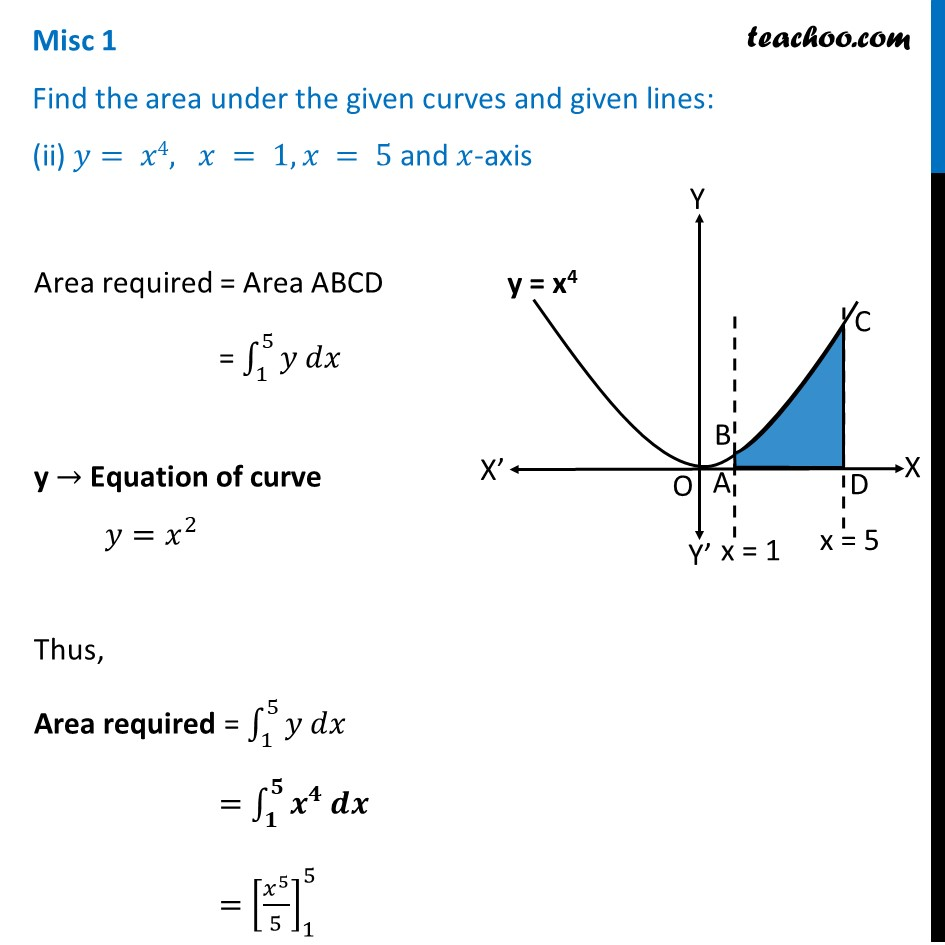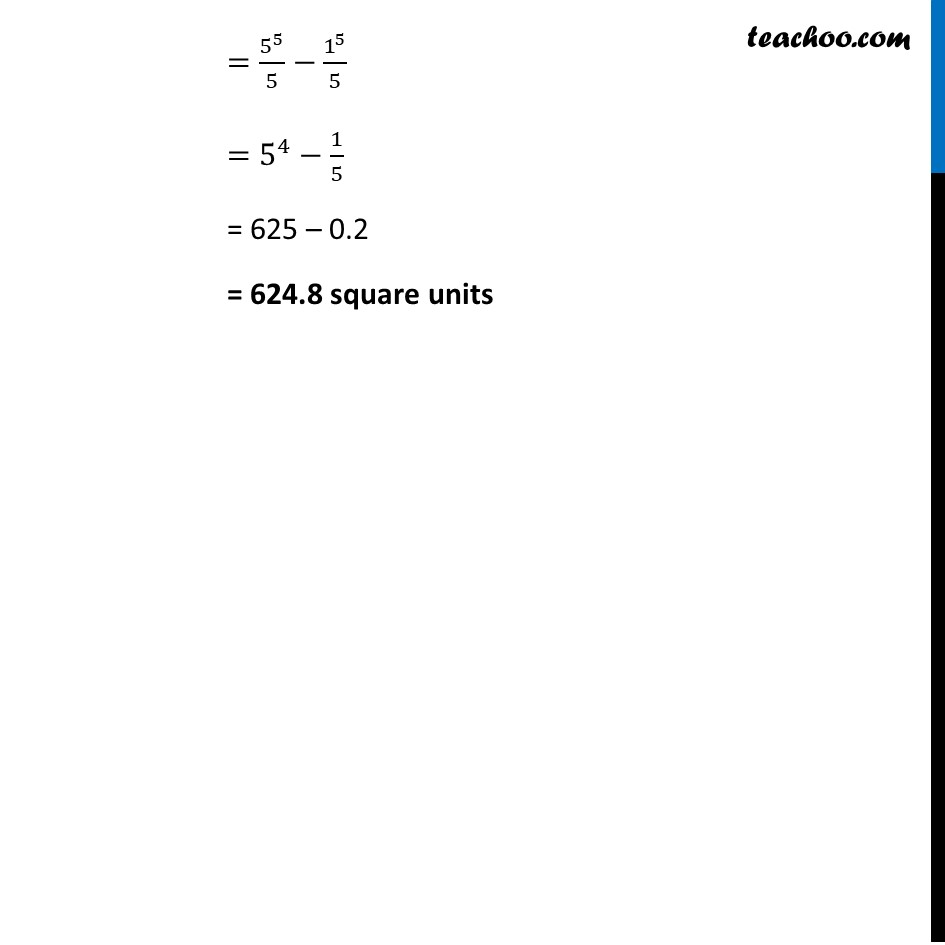Miscellaneous

Chapter 8 Class 12 Application of Integrals
Serial order wiseLearn in your speed, with individual attention - Teachoo Maths 1-on-1 Class

### Transcript

Misc 1 Find the area under the given curves and given lines: (ii) 𝑦= 𝑥4, 𝑥 = 1, 𝑥 = 5 and 𝑥-axisArea required = Area ABCD = ∫_1^5▒〖𝑦 𝑑𝑥〗 y → Equation of curve 𝑦=𝑥^2 Thus, Area required = ∫_1^5▒〖𝑦 𝑑𝑥〗 =∫_𝟏^𝟓▒〖𝒙^𝟒 𝒅𝒙〗 =[𝑥^5/5]_1^5 =5^5/5−1^5/5 =5^4−1/5 = 625 – 0.2 = 624.8 square units